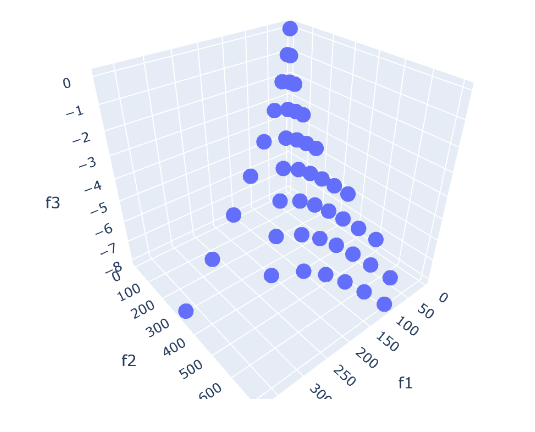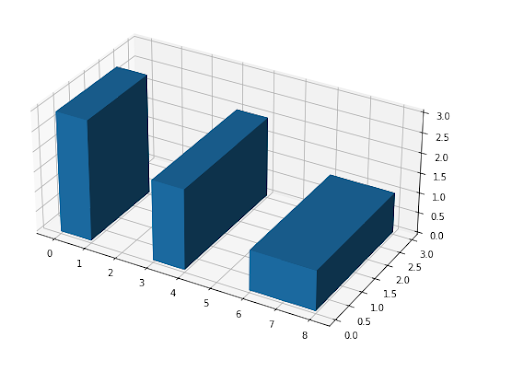## Thursday, October 21, 2021

### A multi-objective nonlinear integer programming problemPicture of the efficient points

In , a small multi-objective non-convex MINLP problem is stated:

Let's see how one could approach a problem like this.

## Saturday, October 16, 2021

### Stacking 3d boxes under rotationBoston custom house tower

Assume we have an infinite collection of 3d boxes of different sizes. As an example let's use :

----     30 PARAMETER boxes  available box sizes

size-x      size-y      size-z

box1           4           6           7
box2           1           2           3
box3           4           5           6
box4          10          12          32


We want to build a tower of boxes such that the base of the box on top is strictly smaller than the underlying box. The "strictly" part is essential. Otherwise, we can just repeat the same type of box ad infinitum. The interesting angle is: we can rotate the boxes. Without loss of generality, we can assume that for all boxes, after rotation, we have: the width ($$x$$) is less than the depth $$(y$$). (This may require a bit of thinking). With this, any box can be placed in just three different ways.3d rotations

The objective is to build a tower that is as tall as possible. In , this problem is solved using a Dynamic Programming algorithm. Here I want to see how this looks using a MIP model.

## Monday, October 11, 2021

### 2d knapsack problem

This post is about what sometimes is called a 2d knapsack problem. It can also be considered as a bin-packing problem. These are famously difficult problems, so let's have a look at a relatively small instance.

So, we have one 2d container of a given size. Furthermore, we have a collection of items: rectangles of a given size, with a value. The goal is to pack items in our container without overlapping each other such that the total value is maximized.

To make things concrete, let's have a look at a small data set (randomly generated):

----     18 PARAMETER data  problem data

width      height   available       value  value/size

k1             20.000       4.000       2.000     338.984       4.237
k2             12.000      17.000       6.000     849.246       4.163
k3             20.000      12.000       2.000     524.022       2.183
k4             16.000       7.000       9.000     263.303       2.351
k5              3.000       6.000       3.000     113.436       6.302
k6             13.000       5.000       3.000     551.072       8.478
k7              4.000       7.000       6.000      86.166       3.077
k8              6.000      18.000       8.000     755.094       6.992
k9             14.000       2.000       7.000     223.516       7.983
k10             9.000      11.000       5.000     369.560       3.733
container      30.000      20.000